• Help with a fluid mechanics assignment on calculating the Molar Volume
• Assistance with getting a Regular Falsi Method Function on a fluid mechanics homework
• The Reynolds Numberapplication by our Online Fluid Mechanics Tutor
• MATLAB Code:

## Help with a fluid mechanics assignment on calculating the Molar Volume

In this fluid mechanics assignment help service, the homework helper has compared the molar volume of Cl2 gas at different pressures obtained by the Ideal Gas Law with those obtained by Redlich-Kwong Equation.

The Ideal Gas Law is stated as follows:

Pv=RT

where

• P is the pressure of the gas,
• v is the volume of the gas
• R is the ideal, or universal, gas constant
• T is the absolute temperature of the gas

The Redlich-Kwong equation is given as:

${{\frac{Pv}{RT}= \frac{v}{v-b}- \frac{a}{RT^{\frac{3}{2}}(v+b)}}}$………………..(1)

where here a and b are: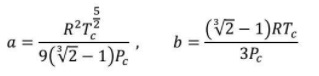where:

Tc is the temperature at the critical point, and

Pc is the pressure at the critical point.

or equivalently as: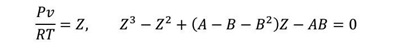………………..(2)

where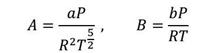Again it required the assignment helper to show that the give two forms of the Redlich-Kwong equation are indeed equivalent

 Parameter Temperature (in K) UniversalGas Constant(in L atm/(K mol) Critical Pressure (in atm) Critical Temperature (in K) Value 293 0.082057 76.1 416.9

Table 1: Values of various parameters used

 Pressure(in atm) 1 1.5 2 2.5 3 5 10 15 25 50 100

Table 2: Given Pressure values

## Assistance with getting a Regular Falsi Method Function on a fluid mechanics homework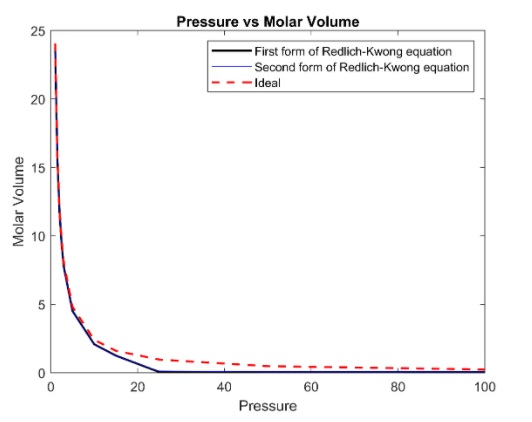Pressure Molar volume (by ideal gas law) Molar volume (by Redlich-Kwong Equation's 1st form) Molar volume (by Redlich-Kwong Equation's 2ndform) 1 24.055 23.765 23.51 1.5 16.037 15.745 11.602 2 12.028 11.73 11.60 2.5 9.62 9.32 9.21 3 9.01 7.72 7.62 5 4.81 4.50 4.44 10 2.4055 2.08 2.04 15 1.60 1.24 1.20 25 0.962 0.0541 0.124 50 0.4811 0.053 0.066 100 0.24 0.053 0.055

We can infer that the Ideal gas law starts to differ from the Redlich-Kwong Equation at higher pressures. This may be attributed to the greater interaction between the molecules due to higher intermolecular forces in case of higher pressure.

The problem also required the fluid mechanics assignment helper to show that equation (1) and (2), which are two forms of Redlich Kwong equation, are the same.

From Equation (1)

${\frac{Pv}{RT}=\frac{v}{v-b}-\frac{a}{(RT^{\frac{3}{2}})(v+b)}}$

Simplifying the RHS

${\frac{Pv}{RT}=\frac{v(RT^{\frac{3}{2}})(v+b)-a(v-b)}{(v-b)(RT^{\frac{3}{2}})(v+b)}}$

Cross Multiplying the denominators and Simplifying

${Pv=\frac{v(RT^{\frac{3}{2}})(v+b)-a(v-b)}{(v-b)(T^{\frac{1}{2}})(v+b)}}$

${Pv=\frac{v(RT^{\frac{3}{2}})(v+b)-a(v-b)}{(v^2-b^2)(T^{\frac{1}{2}})}}$

${pv(v^2-b^2)(T^{\frac{1}{2}})=v(RT{\frac{3}{2}})(v+b)-a(v-b)}$

${PT^{\frac{1}{2}}v^3-PT^{\frac{1}{2}}vb^2=v^2(RT^{\frac{3}{2}})+vb(RT^{\frac{3}{2}})-av+ab}$

${PT^{\frac{1}{2}}v^3-PT^{\frac{1}{2}}vb^2=v^2(RT^{\frac{3}{2}})-vb(RT^{\frac{3}{2}})+av-ab=0}$

Dividing both sides by PT12 and further simplifying

${\frac {PT^{\frac{1}{2}}y^3}{PT{\frac{1}{2}}}-\frac {PT^{\frac{1}{2}}yb^2}{PT{\frac{1}{2}}}-\frac{v^2(RT^{\frac{3}{2}})}{PT^{\frac{1}{2}}}-\frac{vb(RT^{\frac{3}{2}})}{PT^{\frac{1}{2}}}+\frac{av}{PT^{\frac{1}{2}}}-\frac{ab}{PT^{\frac{1}{2}}}=0}$

${v^3-vb^2-\frac{v^2(RT)}{P}-\frac{vbRT}{P}+\frac{av}{PT^{\frac{1}{2}}}-\frac{ab}{PT^{\frac{1}{2}}}=0}$

${v^3-\frac{v^2(RT)}{p}+v(-b^2-\frac{bRT}{p}+\frac{av}{PT^{\frac{1}{2}}})-\frac{ab}{PT^{\frac{1}{2}}}=0}$      ………………. (3)

From Equation (2)

${\frac{Pv}{RT}=Z}$

${A=\frac{aP}{R^2 T^{5/2}}}$

${B=\frac{bP}{RT}}$

${Z^3-Z^2+(A-B-B^2)Z-AB=0}$

${\frac{P^3 y^3}{R^3 T^3}-\frac{P^2 y^2}{R^2 T^2}+\frac{Py}{RT}(\frac{aP}{R^2 T^{\frac{5}{2}}}-\frac{bP}{RT}-\frac{b^2 P^2}{R^2 T^2})-\frac{abP^2}{R^3 T^{\frac{3}{2}}}=0}$

${\frac{R^3 T^3}{P^3}\frac{P^3 v^3}{R^3 T^3}-\frac{R^3 T^3}{P^3}\frac{P^2 v^2}{R^2 T^2}+\frac{R^3 T^3}{P^3}\frac{Pv}{RT}(\frac{aP}{R^2 T^{\frac{5}{2}}}-\frac{bP}{RT}-\frac{b^2 P^2}{R^2 T^2})-\frac{R^3 T^3}{P^3}\frac{abP^2}{R^3T^{\frac{7}{2}}}=0}$

${v^3-\frac{RTv^2}{P}+\frac{R^2T^2v}{P^2}(\frac{aP}{R^2T^{\frac{5}{2}}}-\frac{bP}{RT}-\frac{b^2P^2}{R^2T^2})-\frac{ab}{PT^{\frac{1}{2}}}=0}$

${v^3-\frac{RTv^2}{P}+\frac{R^2T^2v}{P^2}\frac{aP}{R^2T^{\frac{5}{2}}}-\frac{R^2T^2v}{P^2}\frac{bP}{RT}-\frac{R^2T^2v}{P^2}\frac{b^2P^2}{R^2T^2}-\frac{ab}{PT^{\frac{1}{2}}}=0}$

${v^3-\frac{RTv^2}{P}+\frac{v}{P}\frac{a}{T^{\frac{1}{2}}}-v\frac{RT}{P}b-vb^2-\frac{ab}{PT^{\frac{1}{2}}}=0}$

${v^3-\frac{RTv^2}{P}+v(\frac{a}{PT^{\frac{1}{2}}}-\frac{bRT}{P}-b^2)-\frac{ab}{PT^{\frac{1}{2}}}=0}$     ………………. (4)

As we can see, equation (1) upon simplification becomes equations(3), and equation (2) becomes (4), both of which are identical. It is proved that the two forms of Redlich Kwong equation are indeed equivalent

## The Reynolds Numberapplication by our Online Fluid Mechanics Tutor

In this question, the our fluid mechanics tutor has plotted a Moody chart by calculating the Darcy Friction Factor for different values of Reynolds Number ranging from 800 to 250,000,000 and also for different values of relative roughness.

The Darcy friction factor can be calculated for given values Reynolds Number and relative roughness by Colebrook equation

(1)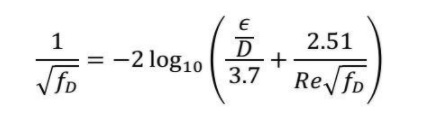fD is the Darcy Friction Factor

${\frac{\varepsilon}{D}}$ is the relative roughness value

Re is Reynolds number for the flow

We are using the Regula Falsi method to find the values of fD corresponding to the given Reynolds Number and relative roughness. This is done by finding the roots of the following equation:

${\frac{1}{\sqrt{f_D}}=-2 \log_{10}(\frac{\frac{\epsilon}{D}}{3.7}+ \frac{2.51}{Re \sqrt f_D})=0}$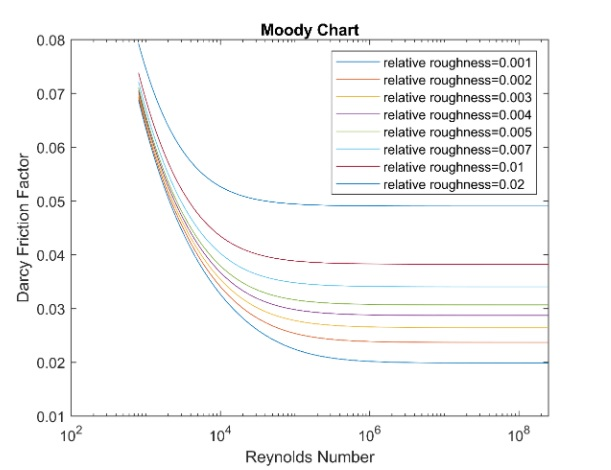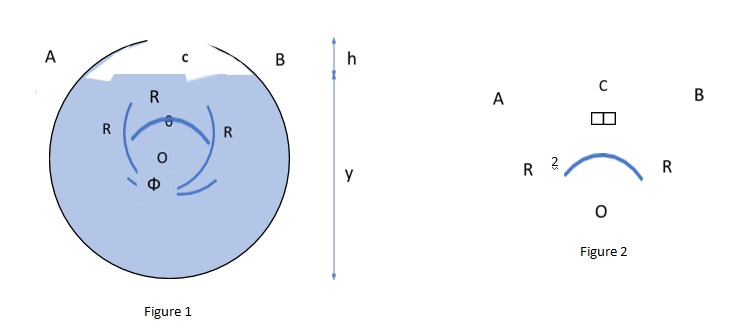Here, we have a pipe in which water has in which water is filled up to depth y in figure 1.

In figure 2, we drop a perpendicular from O to AB to get triangle OAC and OBC

In triangle OAC,

${\cos cos(\frac{\Theta}{2})=\frac{OC}{OA}}$

We know OD = OC +CD=R from figure 1

And CD = h

Therefore OC=R-h

${\cos cos(\frac{\Theta}{2})=\frac{R-h}{R}}$

Therefore

${\frac{\Theta}{2}=(\frac{R-h}{R})}$

Which Implies

${\Theta=2(\frac{R-h}{R})}$

We know, the perimeter of an arc = Radius*angle subtended

Therefore Wetted Perimeter(P)is given by

P=R Φ

We knowΦ=2 π-${\Theta}$ , therefore

${P=R \pi -\Theta}$

We know, wetted area A is given by

A=Area below chord AB

A=Area of sector OAB + are of triangle OAB

A=1/2Angle subtended R2 +1/2base height

${A=(2\pi-\Theta)R^2+\frac{1}{2}AB OC}$

In triangle OAC sin(θ/2)=AC/R

${sin(\frac{\Theta}{2})=\frac{AC}{R}}$

${AC=R sin(\frac{\Theta}{2})}$

Similarly

${OC=R cos(\frac{\Theta}{2})}$

We know AB=2 AC

${A=\frac{1}{2}(2 \pi-\Theta)R^2+\frac{1}{2}2 R sin(\frac{\Theta}{2})Rcos(\frac{\Theta}{2})}$

${A=\frac{1}{2}(2 \pi-\Theta)R^2+\frac{1}{2}R^2 (2sin(\frac{\Theta}{2})cos(\frac{\Theta}{2}))}$

We know

${2sin(\frac{\Theta}{2})cos(\frac{\Theta}{2})=sin(\Theta)}$

Therefore.

${A=\frac{1}{2}(2 \pi-\Theta)R^2+\frac{1}{2}R^2 sin (\Theta)}$

Which implies

${A=\frac{R^2(2 \pi-\Theta+sin(\Theta))}{2}}$

For the numerical, the given values are

 Length (in m) Depth(in m) Radius of pipe(in m) Volumetric flow rate(m3/sec) Acceleration due to gravity(m/sec2) Density of Water(in kg/m3) Reynolds Number Relative Roughness 40 1.8 1.2 12 9.81 997 1500000 0.008

## MATLAB Code:

Main Script:

 Friction Factor Hydraulic Diameter(in m) Average Flow Rate(in m/sec) Head Loss(in m) Pressure Loss(in Pa/m) 0.035245 2.8962 3.2972 0.26972 2638